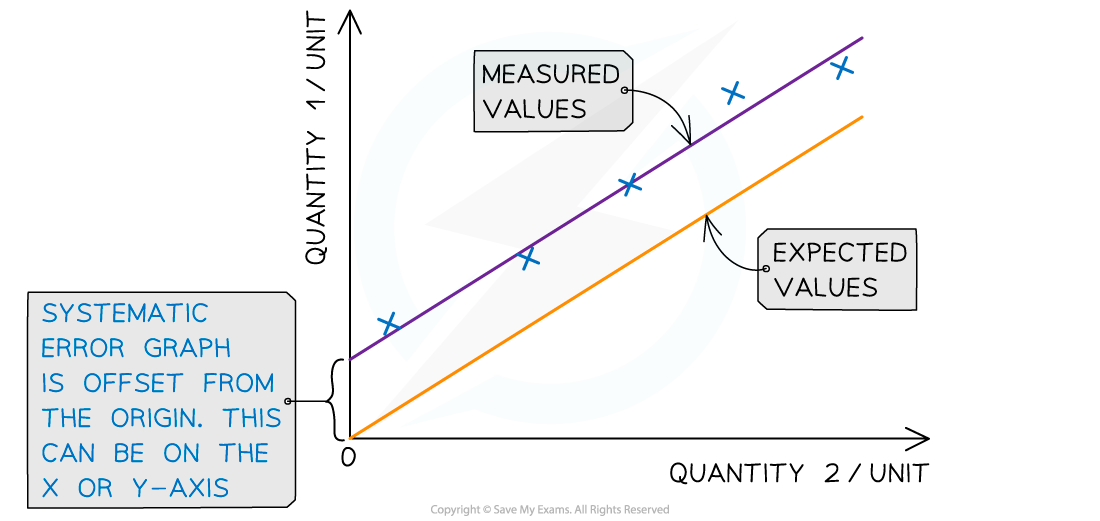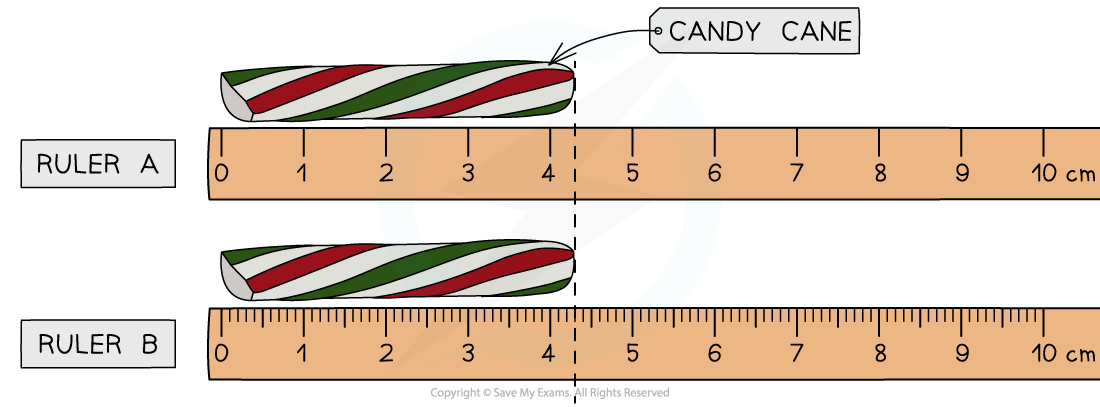# IB DP Physics: SL复习笔记1.2.1 Random & Systematic Errors

### Random & Systematic Errors

• Measurements of quantities are made with the aim of finding the true value of that quantity
• In reality, it is impossible to obtain the true value of any quantity as there will always be a degree of uncertainty
• The uncertainty is an estimate of the difference between a measurement reading and the true value
• The two types of measurement errors that lead to uncertainty are:
• Random errors
• Systematic errors

#### Random Errors

• Random errors cause unpredictable fluctuations in an instrument’s readings as a result of uncontrollable factors, such as environmental conditions
• This affects the precision of the measurements taken, causing a wider spread of results about the mean value
• To reduce random error:
• Repeat measurements several times and calculate an average from them

#### Systematic Errors

• Systematic errors arise from the use of faulty instruments used or from flaws in the experimental method
• This type of error is repeated consistently every time the instrument is used or the method is followed, which affects the accuracy of all readings obtained
• To reduce systematic errors:
• Instruments should be recalibrated, or different instruments should be usedSystematic errors on graphs are shown by the offset of the line from the origin

#### Zero Errors

• This is a type of systematic error which occurs when an instrument gives a reading when the true reading is zero
• For example, a top-ban balance that starts at 2 g instead of 0 g
• To account for zero errors
• Take the difference of the offset from each value
• For example, if a scale starts at 2 g instead of 0 g, a measurement of 50 g would actually be 50 – 2 = 48 g
• The offset could be positive or negative

• When measuring a quantity using an analogue device such as a ruler, the uncertainty in that measured quantity is ±0.5 the smallest measuring interval
• When measuring a quantity using a digital device such as a digital scale or stopwatch, the uncertainty in that measured quantity is ±1 the smallest measuring interval
• Use a more precise device with smaller measuring intervals and therefore less uncertaintyBoth rulers measure the same candy cane, yet Ruler B is more precise than Ruler A due to smaller interval size